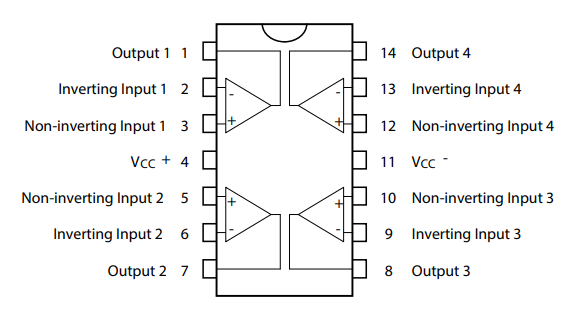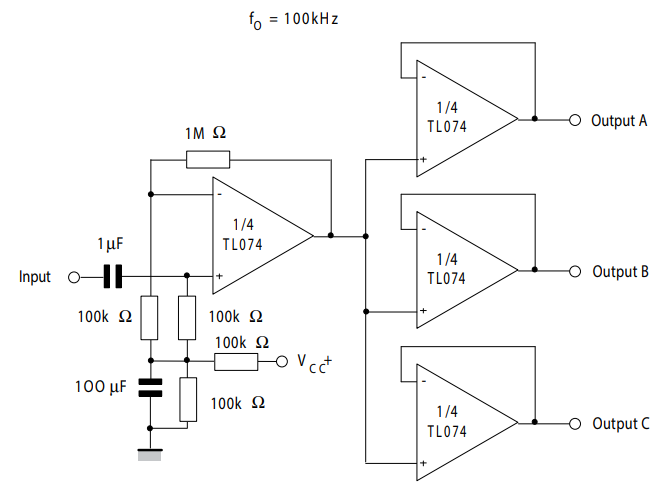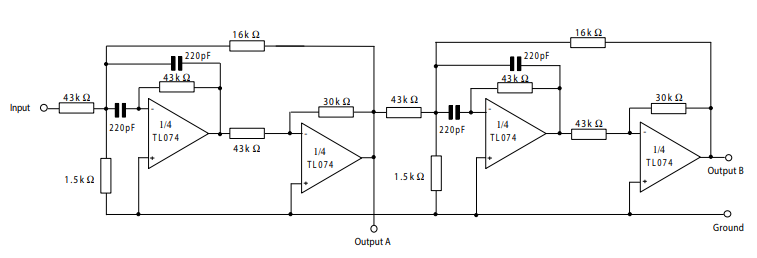TL074 is a JFET-input quad operational amplifier monolithic integrated circuit. This op-amp IC contains four OP-AMPs. It consists of high voltage junction field-effect transistors (JFETs) and Bi-polar junction transistors (BJTs). In addition,  Input bias and bias currents are low. On the contrary,  the slew rate is very high. TL074 is highly recommended for audio pre-amplification as it has low noise and low harmonic distortion. Most importantly, input offset adjustment is possible externally making use of the specific circuits. Furthermore, frequency compensation is possible internally and the operation of TL074 is rendered as latch-up-free. TL071 and TL072 are the single and dual OP-AMP versions of the TL07X family.

## TL074 Op-Amp Pinout Diagram

TL074 comes in 14 pins plastic micro package as DSO8. This diagram shows a  pinout diagram.### TL074 Pin Configuration Description

Firstly, we will begin with a brief description of all the pins.

PINS Details
1 (Out 1-Output 1)  output pin of 1st OP-AMP
2 ( Input 1-inverting Input)  inverting input voltage to 1st OP-AMP
3 ( Input 1- non-Inverting Input)  non-inverting input voltage to 1st OP-AMP
4 (Vcc+)  connect the positive supply voltage
5 ( Input 2- non-Inverting Input)  non-inverting input voltage to 2nd OP-AMP
6 ( Input 2-inverting Input) an inverting input voltage to 2nd OP-AMP
7 (Out 2- Output 2)  output of 2nd OP-AMP
8 (Out 3- Output 3)  output of 3rd OP-AMP
9 ( Input 3-inverting Input)  an inverting input voltage to 3rd OP-AMP
10 ( Input 3- non-Inverting Input) non-inverting input voltage to 3rd OP-AMP
11 (Vcc-) connecting negative supply voltage
12 ( Input 4- non-Inverting Input)  non-inverting input voltage to 4th OP-AMP
13 ( Input 4-inverting Input)  an inverting input voltage to 4th OP-AMP
14 (Out 4- Output 4) output of 4th OP-AMP

## TL074 Electrical Features

The operational features of TL074 are shown as:

Parameters TL074
Input offset voltage (mV) 10
Input bias current (nA) 200
Input Offset Current (nA) 100
Input Common-Mode Voltage Range (V) -12 to +15
Large signal voltage gain (V/mV) 200
Supply  current (mA) 2.5
Common mode rejection ratio (dB) 86
Supply Voltage Rejection Ratio (dB) 86
Supply Voltage (V) 6-36
Slew Rate (V/µs) 13
Output short circuit current (mA) 60
Power Dissipation (mW) 680
Differential Input Voltage (V) ±30

### Equivalents

LM324, TL071, TL072

## How to Use

We need two power supplies to operate TL074. The terminals or the pins used are pins no. 4 and 11. Pin no 4 is for positive power supply. Pin no 11 is for negative power supply. For the first OP-AMP, inverting input is applied at pin 2. Non-inverting is a pin 3 for the second operational amplifier. The output of the first OP-AMP is obtained at pin no. 1.

• For the second OP-AMP, inverting input is applied at the pin. No 6 and non-inverting at pin no. 5.The output of the second OP-AMP is obtained at pin no. 7.
• For the third OP-AMP, inverting input is applied at the pin. No 9 and non-inverting at pin no. 10. The output of the third OP-AMP is obtained at pin no. 8.
• For the fourth OP-AMP, inverting input is applied at the pin. No 13 and non-inverting at pin no. 12. The output of the fourth OP-AMP is obtained at pin no. 14.

## Where to use

### TL074 Example Circuits

One of the uses of TL074 is the audio preamplifier. This is an example circuit utilized for the audio distribution amplifier using TL074.Another example of TL074 as a positive feedback bandpass filter is shown as:## TL074 Applications

TL074 can be used for the construction of the following circuits:

• voltage follower
• Unity gain inverting amplifier
• bilateral current source
•  AC/DC converter
•  instrumentation amplifier
•  square wave generator
•  voltage comparator
• Power supplies
• oscillators
• half-wave rectifiers

## TL074 Practical Examples

In the end,  these are some real-life applications of UA741.

• on our mobile phones for AD conversion
• In Audio amplifiers
• Programmable logic controllers
• Video signal conditioning units
• Processors
•  Sensor Data Accusation
• digital to analog converters in Phone
•  Temperature sensors and controllers
•  Error amplifiers
•  communication circuits
• Mobile phone chargers